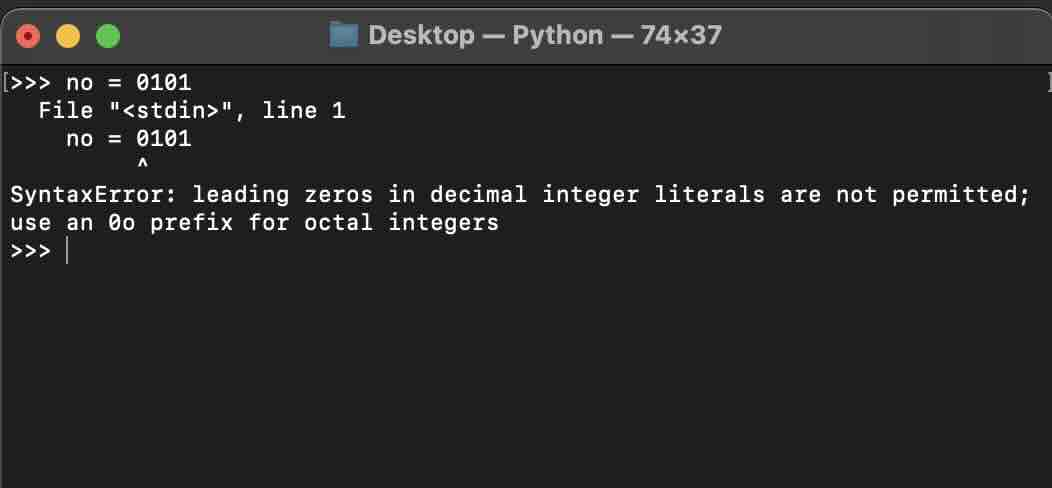# Python: SyntaxError: leading zeros in decimal integer literals are not permitted; use an 0o prefix for octal integersExample:

``````integer_no = 0101

print(integer_no)``````

Error:

``````SyntaxError: leading zeros in decimal integer literals are not permitted;

use an 0o prefix for octal integers``````

## Reason for leading zero SyntaxError

In Python Programming, leading zeroes to a number value is not allowed, as having a leading zero, the number (assumed to be decimal - base 10) is considered to be an Oactal number (to the base 8)

But even to represent a number of octal (base 8( type, you have to add prefix 0o (Numeric 0 followed by lowercase letter o)

## Solution/Fix

There could be one of these solutions based on what you are trying to achieve.

### 1. If the number is a decimal number

Simply remove the zero prefixed to the number.

Example:

``decimal_no = 101``

### 2. If the number is an octal number

Add a lowercase letter 0o before the number.

Example:

``octal_no = 0o101``

### 3. If the value is to be a String

Surround the number with either a single or double quote.

Example:

``string = "0101"``
-

### Facing issues? Have Questions? Post them here! I am happy to answer!Author Info:

Rakesh is a seasoned developer with over 10 years of experience in web and app development, and a deep knowledge of operating systems. Author of insightful How-To articles for Code2care.